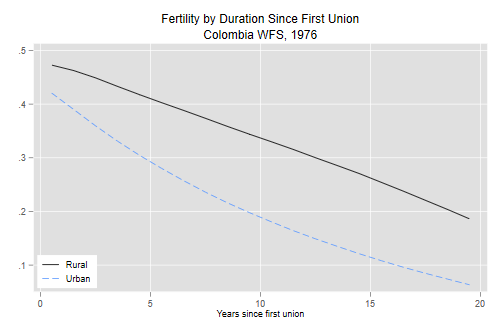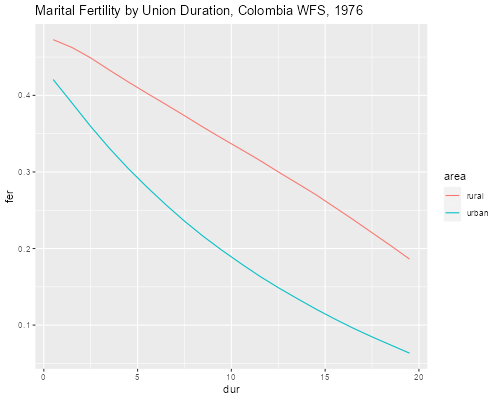Germán Rodríguez
Demographic Methods Princeton University## The Page Model of Marital Fertility

We apply Page’s model of marital fertility by age and duration to study urban-rural differentials in Colombia, following essentially the procedures in Rodríguez and Cleland (1980).

We will work with an extract from the 1976 WFS that has the dates of R’s birth, first union and interview, the birth history, and type of place of residence.

```. use https://grodri.github.io/datasets/cofertx, clear
(COSR02 extract)
```
```> library(haven)
```

### Events and Exposure

We will focus on fertility in the three-years before the interview. A woman starts contributing events and exposure 36 months before the interview or when she enters a first union, whichever occurs later. We create the variables `bot` and `top` to mark the start and end of exposure.

```. gen bot = v007 - 36

. replace bot = m012 if m012 > bot

. gen top = v007 - 1

. drop if bot >= top // no in-union exposure
(2,088 observations deleted)
```
```> library(dplyr)
> co <- mutate(co,
+   bot = ifelse(m012 > v007 - 36, m012, v007 - 36),
+   top = v007 - 1) |>
+ filter(bot < top)
```

The next step is to count births in the window, occurring between `bot` and `top` (the month before the interview). The WFS coded the dates of birth of up to 24 children in variables called `b012 b022 b032 ... b242`. We loop by variable and use a local macro zj`paste()`to construct the names with zero-padded birth numbers.

```. gen births = 0

. forvalues j = 1/24 {
2.   local zj = "`j'"
3.   if `j' < 10 local zj = "0`j'"
4.   quietly replace births = births + 1 if b`zj'2 >= bot & b`zj'2 <= top
5. }
```
```> co\$births <- 0
> for(j in 1:24) {
+   name = paste(ifelse(j < 10, "b0","b"), j, "2", sep="")
+   co\$births <- co\$births + (co[,name] >= co\$bot & co[,name] <= co\$top)
+ }
```

Each woman is exposed for exactly 36 months unless she married in the last three years, in which case we take the difference between the dates of marriage and interview. (One could count only half a month of exposure for the calendar month of marriage, but I will ignore this refinement, partly because we do count all births in that calendar month anyway.) We also need the midpoint of the window, so we can compute age and duration since first union at that time.

```. gen expo = (top - bot + 1)/12

. gen mid = (bot + top)/2

. gen age = (mid - v008)/12

. gen dur = (mid - m012)/12
```
```> co <- mutate(co,
+   expo = (top - bot + 1)/12,
+   mid = (bot + top)/2,
+   age = (mid - v008)/12,
+   dur = (mid - m012)/12)
```

### Regression Analysis

Next we use our handy function to compute natural fertility, and calculate an offset equal to the log of natural fertility times exposure time

```. egen nf = natfer(age)

. gen os = log(nf * expo)
```
```> library(nuptfer)
> co <- mutate(co,
+   nf = natfer(age),
+   os = log(nf * expo))
```

All that remains is to fit the model using two terms to represent the effects of urban residence: a dummy variable for the spacing effect and an interaction with duration for the limiting effect (which I create to get a simpler name).

```. gen urban = v702 == 1

. gen urbanXdur = urban * dur

. poisson births dur urban urbanXdur, offset(os)

Iteration 0:   log likelihood = -2881.5137
Iteration 1:   log likelihood = -2878.5968
Iteration 2:   log likelihood =  -2878.587
Iteration 3:   log likelihood =  -2878.587

Poisson regression                                      Number of obs =  3,290
LR chi2(3)    = 319.95
Prob > chi2   = 0.0000
Log likelihood = -2878.587                              Pseudo R2     = 0.0526

─────────────┬────────────────────────────────────────────────────────────────
births │ Coefficient  Std. err.      z    P>|z|     [95% conf. interval]
─────────────┼────────────────────────────────────────────────────────────────
dur │  -.0254673   .0050656    -5.03   0.000    -.0353958   -.0155388
urban │  -.0968007   .0738112    -1.31   0.190     -.241468    .0478666
urbanXdur │  -.0466704   .0074747    -6.24   0.000    -.0613205   -.0320203
_cons │   .0077587   .0559107     0.14   0.890    -.1018243    .1173417
os │          1  (offset)
─────────────┴────────────────────────────────────────────────────────────────
```
```> co <- mutate(co, urban = as.numeric(v702 == 1)) # 1=urban
> m <- glm(births ~ dur * urban + offset(os),
+   data = co, family = poisson)
> coef(summary(m))
Estimate  Std. Error   z value     Pr(>|z|)
(Intercept)  0.007758676 0.055910722  0.138769 8.896327e-01
dur         -0.025467276 0.005065645 -5.027449 4.970466e-07
urban       -0.096800703 0.073811211 -1.311463 1.897012e-01
dur:urban   -0.046670381 0.007474684 -6.243794 4.270837e-10
```

The results show a spacing parameter of 0.008 and a limiting parameter of -0.025 for rural areas. Urban areas have essentially the same level of natural fertility (the difference of -0.097 is not significant), but significantly higher levels of limiting behavior (the difference of -0.467 is highly significant).

### Translation into Rates

We can translate these results into rates that may be easier to present. First we compute the mean age at first union of our sample, and notice that urban women married about 10 months later than rural women.

```. gen afu = (m012 - v008)/12

. tabstat afu, stat(mean) by (urban)

Summary for variables: afu
Group variable: urban

urban │      Mean
─────────┼──────────
0 │  19.05664
1 │  19.89004
─────────┼──────────
Total │  19.59265
─────────┴──────────
```
```> co <- mutate(co, afu = (m012 - v008)/12)
> group_by(co, urban) |> summarize( mean = mean(afu))
# A tibble: 2 × 2
urban  mean
<dbl> <dbl>
1     0  19.1
2     1  19.9
```

We then predict fertility at the mid-point of each year since union, from 0.5 to 19.5, for women entering their first union at these average ages, and sum the rates for the first 20 years in union:

```. gen d = _n - 0.5 in 1/20
(3,270 missing values generated)

. egen nfr = natfer(19.06 + d) in 1/20

. egen nfu = natfer(19.89 + d) in 1/20

. gen fr = nfr * exp(_b[_cons] + _b[dur]*d)
(3,270 missing values generated)

. gen fu = nfu * exp(_b[_cons] + _b[urban] + (_b[dur] + _b[urbanXdur]) *d)
(3,270 missing values generated)

. gen Fu = sum(fu) in 1/20
(3,270 missing values generated)

. gen Fr = sum(fr) in 1/20
(3,270 missing values generated)

. list d Fu Fr in 20

┌──────────────────────────────┐
│    d          Fu          Fr │
├──────────────────────────────┤
20. │ 19.5   4.1681153   6.7047734 │
└──────────────────────────────┘
```
```> d <- 1:20 - 0.5
> nf <- c(natfer(19.06 + d), natfer(19.89 + d))
> nd <- data.frame(
+   urban = rep(c(0,1), c(20,20)),
+   dur = d,
+   os = log(nf))
> nd <- mutate(nd,
+   fer = exp(predict(m, newdata = nd)))
> group_by(nd, urban) |> summarize( tmfr = sum(fer) )
# A tibble: 2 × 2
urban  tmfr
<dbl> <dbl>
1     0  6.70
2     1  4.17
```

The urban and rural total marital fertility rates are 4.17 and 6.70, a difference of two and a half children 20 years after the first union.

Finally we plot duration specific rates at mean ages of entry into union:

```. line fr fu d, lpat(solid dash) ///
>   xtitle(Years since first union) ///
>   title("Fertility by Duration Since First Union") ///
>   subtitle("Colombia WFS, 1976") ///
>   legend(order(1 "Rural" 2 "Urban") ring(0) pos(7))

. graph export comarfer.png, width(500) replace
file comarfer.png saved as PNG format
``````> library(ggplot2)
> nd <- mutate(nd, area = factor(urban, labels = c("rural","urban")))
> ggplot(nd, aes(dur, fer, color=area)) + geom_line() +
+   ggtitle("Marital Fertility by Union Duration, Colombia WFS, 1976")
> ggsave("comarferr.png", width = 500/72, height = 400/72, dpi = 72)
```We see how fertility declines more steeply with duration of union in urban areas.

